# Bessel interpolation formula

A formula which is defined as half the sum of the Gauss formula (cf. Gauss interpolation formula) for forward interpolation on the nodes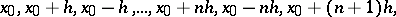at the point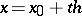: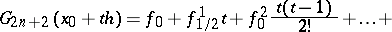(1)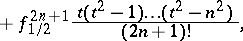and the Gauss formula of the same order for backward interpolation with respect to the node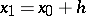, i.e. with respect to the population of nodes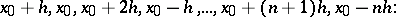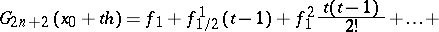(2)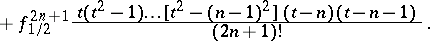Putting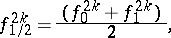Bessel's interpolation formula assumes the form (, ):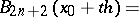(3)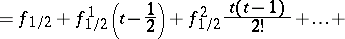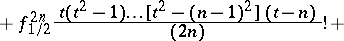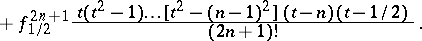Bessel's interpolation formula has certain advantages over Gauss' formulas (1), (2); in particular, if the interpolation is at the middle of the segment, i.e. at, all coefficients at the differences of odd orders vanish. If the last term on the right-hand side of (3) is omitted, the polynomial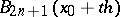, which is not a proper interpolation polynomial (it coincides with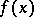only in thenodes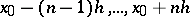), represents a better estimate of the residual term (cf. Interpolation formula) than the interpolation polynomial of the same degree. Thus, for instance, if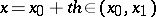, the estimate of the last term using the polynomial which is most frequently employed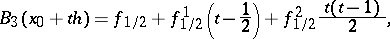written with respect to the nodes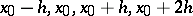, is almost 8 times better than that of the interpolation polynomial written with respect to the nodes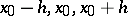or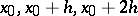().